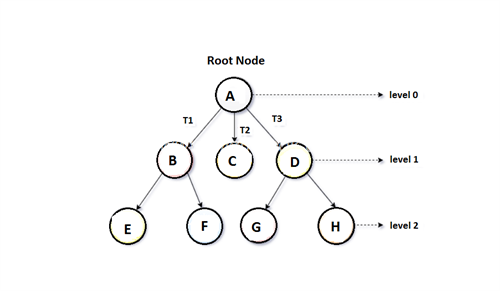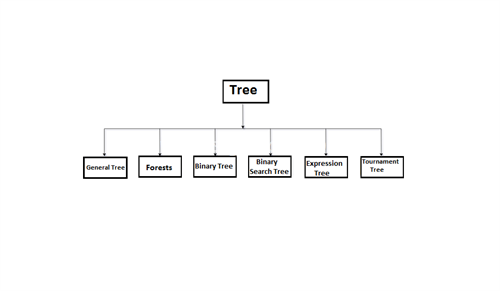Total available pages count: 55
Subject - Java Technologies

## Tree

A tree is a recursive type of non-linear data structure that contains the set of one or more data nodes where one node designated as a toot of tree while the remaining node is known as children of the root.

Generally, Node can have any number of child nodes but it may have only a single parent. the node of the tree either maintains a parent-child relationship between them. The node other than the root node is divided into a non-empty set where each one of them is called a subtree.## Terminology

1. Root node - The root node is the topmost node in the hierarchy. It is known as a parent node
2. Sub Tree - As per the picture, T1, T2 & T3 are known as a subtree of the root node
3. Leaf node - It is a bottom-most node in a tree hierarchy, there are no numbers of leaf nodes available in the general tree
4. Path - The chain of consecutive edges is known as a path. E.g.  A->B->E
5. Ancestor node - It is any Predecessor node on the path from the root to the same node. e.g., node F has ancestor B & A
6. Degree - The degree of a node equals the number of child nodes
7. Level number - Every node of the tree is assigned to a level number in a way that each node is available at one level higher than the parent node
``````/*Static epresentation of tree */
#define MAXNODE 500
Struct treenode
{
int root;
int father;
int son;
int next;
}
Struct treenode
{
int root;
Struct treenode *father;
Struct treenode *son;
Struct treenode *next;
}``````

## Tree Data Structure :## Binary Tree

A binary tree is a generic type of non-linear data structure. In which each node has at most two child nodes. Generally, binary tree divided into three disjoint subsets:

• Root
• Left child
• Right child

## Traversal of Binary Tree :

Pre-order traversal
Parent root first then traverse into left & right subtree. the procedure can be applied to each subtree of the tree recursively

In order Traversal
Traverse first the left subtree, then traverse root & right subtree.

Post-Order Traversal
Traverse left subtree, then traverse right subtree & root.